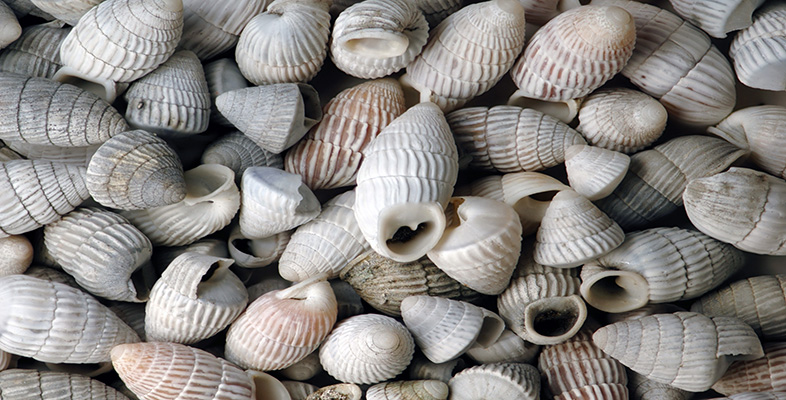Maths everywhere

This free course is available to start right now. Review the full course description and key learning outcomes and create an account and enrol if you want a free statement of participation.

Free course

# Conclusion

This course introduced the varied components that you will use to learn mathematics: a calculator, reader articles, audio and video clips. You have mainly been working from this course's text and the Calculator Book.

A number of activities involved communicating your ideas: the meaning of mathematical terms; where you can ‘see’ mathematics in everyday settings; reviewing how you have studied this material. Writing is one aspect of communication which is central to learning. So it makes sense for you to think about and develop your skills in this area.

Part of learning mathematics is to learn to speak and think like a mathematician: becoming more aware of and fluent with the language of mathematics.

As you have now completed this course of work, take a few minutes to go through the list of outcomes and think about what you have achieved.

## Outcomes

You should now:

• be able to describe your view of what mathematics is (Section 1);

• have begun to recognize different types of written mathematics and developed your skill at reading it (Activities 6–9);

• be able to tackle mathematical problems using a calculator (Calculator Book, Chapter 1);

• be able to use your calculator with understanding for basic arithmetic, percentages, square roots, reciprocals and powers (Calculator Book, Sections 1.2, 1.4, 1.5);

• be able to express and interpret numbers in scientific notation, both in writing and on your calculator (Calculator Book, Section 1.6);

• be able to give some examples of common mathematical ‘doing–undoing’ pairs of operations (Calculator Book, Section 1.7);

• be more attuned to noticing mathematical questions arising from the world around you (Activities 3, 4, 10 and 11);

• have increased experience in working from video and audiotape as part of your mathematical learning (Activities 4, 11 and 19);

• have organized and planned your study (Activities 12–19).

MU120_1